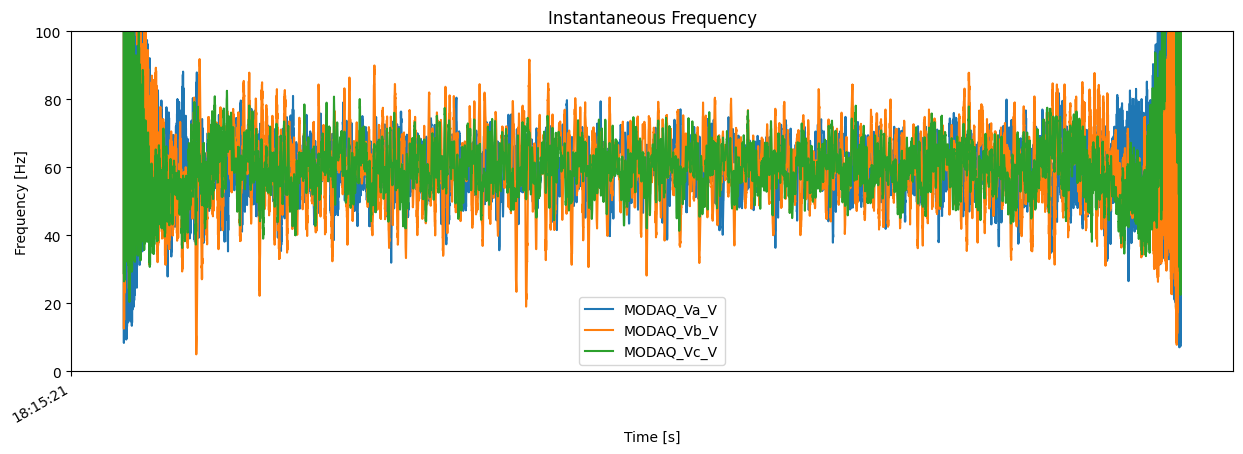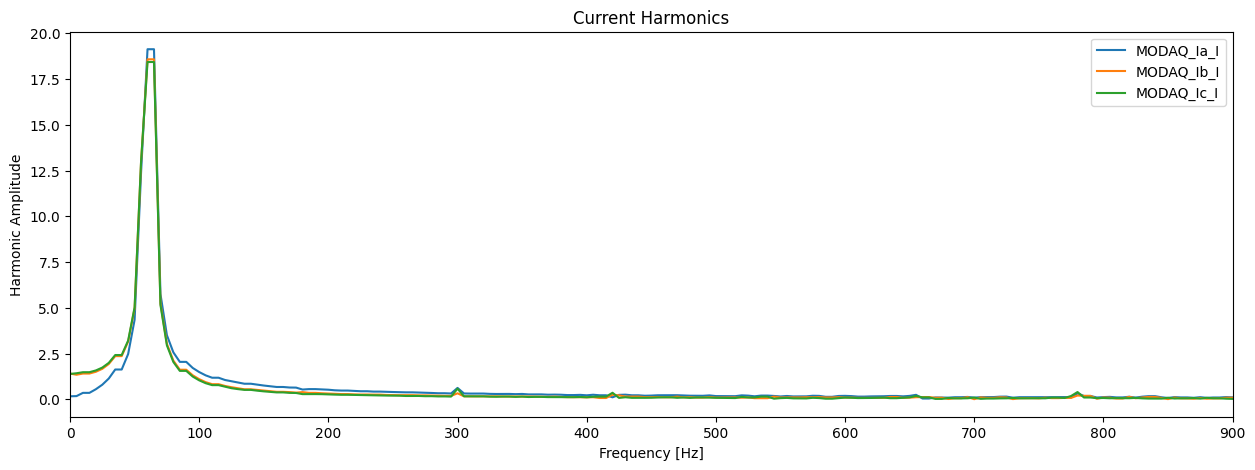# MHKiT Power Module

The following example uses the MHKiT power module to compute power, instantaneous frequency, and harmonics from time-series of voltage and current.

Start by importing the necessary python packages and MHKiT module.

:

import numpy as np
import pandas as pd
from mhkit import power, utils


We will begin by reading in time-series data of measured three phase (a, b, and c) voltage and current.

Note: IEC TS 62600-30 requires power quality assessments on a minimum of 10 minute time-series data, but for this example a shorter time-series is used.

:

# Read in time-series data of voltage (V) and current (I)
# Convert the time index to type "datetime"
power_data.index=pd.to_datetime(power_data.index)
# Display the data

:

MODAQ_Va_V MODAQ_Vb_V MODAQ_Vc_V MODAQ_Ia_I MODAQ_Ib_I MODAQ_Ic_I
Time_UTC
2020-02-24 18:15:21.499998208 10652.764496 -8499.446320 -1850.166138 -23.213654 19.219666 4.023438
2020-02-24 18:15:21.500018208 10691.122620 -8428.702576 -1927.626526 -23.404846 19.181671 4.289856
2020-02-24 18:15:21.500038209 10732.961151 -8364.997162 -2001.269318 -23.493042 19.033966 4.478912
2020-02-24 18:15:21.500058210 10775.892639 -8304.582245 -2071.241791 -23.680115 18.917847 4.858246
2020-02-24 18:15:21.500078210 10818.297028 -8248.074066 -2138.016785 -23.737946 18.702087 5.092468

## Power Characteristics

The power.characteristics submodule is used to compute quantities of interest from voltage and current time-series. In this example, we will calculate active AC power and instantneous frequency.

### Active AC Power

To compute the active AC power, the user must provide a power_factor for the efficency of the device.

:

# First seperate the voltage and current time-series into seperate dataFrames
voltage = power_data[['MODAQ_Va_V', 'MODAQ_Vb_V', 'MODAQ_Vc_V']]
current = power_data[['MODAQ_Ia_I','MODAQ_Ib_I','MODAQ_Ic_I']]

# Set the power factor for the system
power_factor = 0.96

# Compute the instantaneous AC power in watts
ac_power = power.characteristics.ac_power_three_phase(voltage, current, power_factor)
# Display the result
ac_power.Power.plot(figsize=(15,5),title='AC Power').set(xlabel='Time',ylabel='Power [W]');### Instantaneous Frequency

Calculate the instantaneous frequency of the voltage time-series using the 3 phase voltage measurements. The time-varying instantaneous frequency is a required metric for power quality assessments.

:

# Compute the instantaneous frequency
inst_freq = power.characteristics.instantaneous_frequency(voltage)

# Display the result
inst_freq.plot(figsize=(15,5), ylim=(0,100),
title='Instantaneous Frequency').set(xlabel='Time [s]',
ylabel='Frequency [Hz]');

:

MODAQ_Va_V MODAQ_Vb_V MODAQ_Vc_V
1 902.215367 1218.092331 433.063162
2 8.499136 12.753006 28.996487
3 509.904722 671.800108 268.237845
4 10.176332 15.101179 26.504936
5 399.622022 524.697779 217.596577## Power Quality

The power.quality module can be used to compute harmonics of current. and voltage and current distortions following IEC/TS 62600-30 and IEC/TS 61000-4-7. Harmonics and harmonic distortion are required as part of a power quality assessment and characterize the stability of the produced power.

:

# Set the nominal sampling frequency
sample_freq = 50000   #[Hz]

# Set the frequency of the grid the device would be conected to
grid_freq = 60        #[Hz]

# Set the rated current of the device
rated_current = 18.8  # [Amps]

# Calculate the harmonics
harmonics = power.quality.harmonics(current, sample_freq, grid_freq)

# Plot the results
harmonics.plot(figsize=(15,5),xlim=(0,900),
title='Current Harmonics').set(ylabel='Harmonic Amplitude',
xlabel='Frequency [Hz]');### Harmonic Subgroups

The harmonic subgroups calculations are based on IEC/TS 62600-30. We can calculate them using our grid frequency and harmonics.

:

# Calcualte Harmonic Subgroups
h_s = power.quality.harmonic_subgroups(harmonics,grid_freq)
# Display the results

:

MODAQ_Ia_I MODAQ_Ib_I MODAQ_Ic_I
0 0.247401 1.948879 1.991755
60 29.761108 29.305038 29.127020
120 1.870176 1.291483 1.206478
180 1.007562 0.648012 0.535090
240 0.727466 0.437456 0.370414

### Total Harmonic Current Distortion

Compute the THCD from harmonic subgroups and rated current for the device

:

#Finally we can compute the total harmonic current distortion as a percentage
THCD=power.quality.total_harmonic_current_distortion(h_s,rated_current)
THCD

:

MODAQ_Ia_I MODAQ_Ib_I MODAQ_Ic_I
THCD 8.972511 6.096617 5.929491